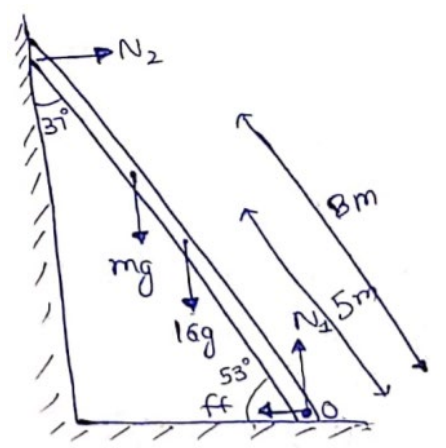# Solve the following :

Question:

Suppose the friction coefficient between the ground and the ladder of the previous problem is $0.540 .$ Find the maximum weight of a mechanic who could go up and do the work from the same position of the ladder.

Solution:Here,$\mu=0.54$

Translatory Equilibrium

$N_{1}=m g+16 g{ }_{-(i)}$

$N_{2}=f f=\mu N_{1}-$ (ii)

$N_{2}\left(10 \sin 53^{\circ}\right)=m g(8 \cos 53)+16 g \cos 53^{\circ}$-(iii)
$m=44 \mathrm{~kg}$